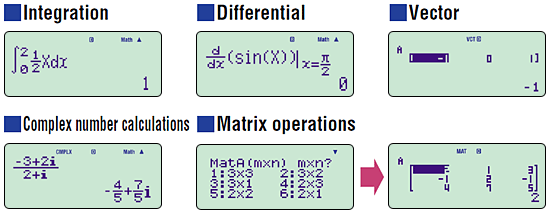# fx-570ES

Close

• The colors may differ slightly from the original.

## fx-570ES

Past Model

10 + 2 digits
10-digit mantissa + 2-digit exponential display.

## Features

• Fraction calculations
• Combination and permutation
• Statistics (List-based STAT data editor, standard deviation, regression analysis)
• 7 variable memories
• Table function
• Comes with new slide-on hard case

### fx-82ES/85ES/350ES functions, in addition to:

• Equation calcuｌations
• Intergration/differential calculations
• Matrix calculations
• Vector calculations
• Complex number calculations
• CALC function
• SOLVE function
• Base- n calculation## Specifications

•Natural textbook display Display expression same as textbook.

•10 + 2 digits 10-digit mantissa + 2-digit exponential display.

•Dot matrix display High-resolution screen provides beautiful looking graphs every time.

•Regular percent Regular percentage calculations.

•Multi-replay Quick and easy recall of previously executed formulas for editing and re-execution.

•Plastic keys Designed and engineered for easy operation.

•List based STAT-data editor Viewing and editing of input data in list format, showing data groups (x-data, y-data, frequency) and surrounding data.

### Size of case / Total weight

• Dimensions (D × W × H) : 161×80×14mm
• Weight : 110g